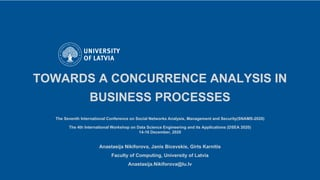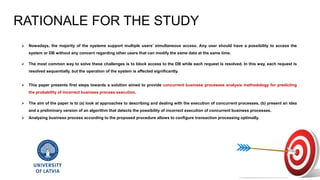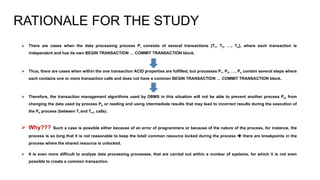Seu SlideShare está sendo baixado. ×

# Towards a Concurrence Analysis in Business Processes

Anúncio
Anúncio
Anúncio
Anúncio
Anúncio
Anúncio
Anúncio
Anúncio
Anúncio
Anúncio
AnúncioCarregando em…3
×

1 de 12 Anúncio

# Towards a Concurrence Analysis in Business Processes

This presentation is a supplementary material for the following article -> Nikiforova, A., Bicevskis, J., & Karnitis, G. (2020, December). Towards a Concurrence Analysis in Business Processes. In 2020 Seventh International Conference on Social Networks Analysis, Management and Security (SNAMS) (pp. 1-6). IEEE.
This paper presents first steps towards a solution aimed to provide concurrent business processes analysis methodology for predicting the probability of incorrect business process execution. The aim of the paper is to (a) look at approaches to describing and dealing with the execution of concurrent processes, mainly focusing on the transaction mechanisms in database management systems, (b) present an idea and a preliminary version of an algorithm that detects the possibility of incorrect execution of concurrent business processes. Analyzing business process according to the proposed procedure allows to configure transaction processing optimally.

This presentation is a supplementary material for the following article -> Nikiforova, A., Bicevskis, J., & Karnitis, G. (2020, December). Towards a Concurrence Analysis in Business Processes. In 2020 Seventh International Conference on Social Networks Analysis, Management and Security (SNAMS) (pp. 1-6). IEEE.
This paper presents first steps towards a solution aimed to provide concurrent business processes analysis methodology for predicting the probability of incorrect business process execution. The aim of the paper is to (a) look at approaches to describing and dealing with the execution of concurrent processes, mainly focusing on the transaction mechanisms in database management systems, (b) present an idea and a preliminary version of an algorithm that detects the possibility of incorrect execution of concurrent business processes. Analyzing business process according to the proposed procedure allows to configure transaction processing optimally.

Anúncio
Anúncio

### Towards a Concurrence Analysis in Business Processes

1. 1. TOWARDS A CONCURRENCE ANALYSIS IN BUSINESS PROCESSES The Seventh International Conference on Social Networks Analysis, Management and Security(SNAMS-2020) The 4th International Workshop on Data Science Engineering and its Applications (DSEA 2020) 14-16 December, 2020 Anastasija Nikiforova, Janis Bicevskis, Girts Karnitis Faculty of Computing, University of Latvia Anastasija.Nikiforova@lu.lv
2. 2.  Nowadays, the majority of the systems support multiple users’ simultaneous access. Any user should have a possibility to access the system or DB without any concern regarding other users that can modify the same data at the same time.  The most common way to solve these challenges is to block access to the DB while each request is resolved. In this way, each request is resolved sequentially, but the operation of the system is affected significantly.  This paper presents first steps towards a solution aimed to provide concurrent business processes analysis methodology for predicting the probability of incorrect business process execution.  The aim of the paper is to (a) look at approaches to describing and dealing with the execution of concurrent processes, (b) present an idea and a preliminary version of an algorithm that detects the possibility of incorrect execution of concurrent business processes.  Analyzing business process according to the proposed procedure allows to configure transaction processing optimally. RATIONALE FOR THE STUDY
3. 3.  There are cases when the data processing process Pi consists of several transactions {T1, T2, …, Tn}, where each transaction is independent and has its own BEGIN TRANSACTION … COMMIT TRANSACTION block.  Thus, there are cases when within the one transaction ACID properties are fulfilled, but processes P1, P2, …, Pn contain several steps where each contains one or more transaction calls and does not have a common BEGIN TRANSACTION … COMMIT TRANSACTION block.  Therefore, the transaction management algorithms used by DBMS in this situation will not be able to prevent another process Pm from changing the data used by process Pk or reading and using intermediate results that may lead to incorrect results during the execution of the Pk process (between Ti and Ti+1 calls).  Why??? Such a case is possible either because of an error of programmers or because of the nature of the process, for instance, the process is so long that it is not reasonable to keep the total/ common resource locked during the process  there are breakpoints in the process where the shared resource is unlocked.  It is even more difficult to analyze data processing processes, that are carried out within a number of systems, for which it is not even possible to create a common transaction. RATIONALE FOR THE STUDY
4. 4.  The study proposes an algorithm for a business process that uses a transaction mechanism, analysis that aims to determine the probability of incorrect concurrent execution of multiple processes.  Main parts: 1) a modeling language called CPL-1 (Concurrent Process Language) that uses the transaction mechanism, 2) an algorithm that, for every two processes defined in CPL-1, determines the probability of an incorrect execution of concurrent processes A BRIEF OVERVIEW OF THE STUDY
5. 5.  programs defined in CPL-1 can use local variables only,  variable can store a real number,  multiple variables can form a logical and numerical expressions,  numeric expressions can have only add- and subtraction operations, WHY???*  programs can use operators assigning value to variable. *if all arithmetic operations and complex functions are allowed to be applied, the conditions of scenarios may contain inequality systems that cannot be resolved  the nature of these operations is limited at this stage, to ensure systems to be solved are linear and easy to analyze, therefore, it is possible to find a solution, if any, or to prove that the solution does not exist. THE PROPOSED COMPUTING SYSTEM processes transactions input data processor =
6. 6. START PROCESS … COMMIT PROCESS BEGIN TRANSACTION … COMMIT TRANSACTION READ(x, R) WRITE(x, R), which suppose read/ write of variable x value to the global resource R IF L THEN BLOCK1 <ELSE BLOCK2> ENDIF, where block can contain one or more commands, for instance: y = EXPR(x1, x2, .., xn), where EXPR is linear expression, x1, x2, .., xn are arguments and y – is a result. CPL-1 does not suppose dealing with cycles* - the programme contains only paths of finite length and the number of concurrent execution scenarios for multiple processes is also finite  for each program defined in CPL-1, the finite scenario tree can be created where each scenario will be in the form of P1(T1>T2(a,b))>P2(T1>T2(y,z)), where a..z are commands to be executed. *The inability to allow cycles is due to the fact that it is not possible to create a complete test set (CTS) for programmes containing cycles and two-way counters. CPL-1: ALLOWED CONSTRUCTIONS
7. 7. The concurrent execution of business processes that uses a transaction mechanism is affected by the order of the execution of multiple individual transactions. This results in two sets of process execution scenarios: (1) concurrent (C) - a set to be analyzed, (2) serial (S) - a set against which the first set to be analyzed. There may be several different, but correct results - depending on the order of execution, one of the possible correct results is achieved a criterion for any process and any input data correctness is the result obtained by one of the serial processes CONCURRENT PROCESSES AND THEIR EXECUTION CORRECTNESS time dimension P1 P2 time dimension P1 P2 time dimension P1 P2 Concurrent execution of P1 and P2 Serial execution of P1 and P2
8. 8. Step I: creating a tree of possible scenarios Step II: executing scenarios symbolically  defining the feasibility conditions Step III: comparing serial and concurrent execution conditions and results  identifying incorrect execution ✓the highest level of concurrency may be granted !!! Incorrect execution detected  redo the implementation of the business process !!! NOT EQUAL !!! EQUAL THE PROPOSED ALGORITHM
9. 9. Payments p1 and p2 must be made from the bank account r (resource r). It is carried out by two processes Payment (p1, r) and Payment (p2, r) START PROCESS Payment(p, globalR) BEGIN TRANSACTION T1 2 READ (x,r) COMMIT TRANSACTION T1 BEGIN TRANSACTION T2 3 IF p<=x THEN 4 WRITE (x-p,r) 5 ENDIF COMMIT TRANSACTION T2 END PROCESS CPL-1: EXAMPLE Path condition result P1(T1>T2(3,5))=>P2(T1>T2(3,5)) (v1>r)&(v2>r) r P1(T1>T2(3,4,5))=>P2(T1>T2(3,5)) (v1<=r)&(v2>r-v1) r-v1 P1(T1>T2(3,5))=>P2(T1>T2(3,4,5)) (v1>r)&(v2<=r) r-v2 P1(T1>T2(3,4,5))=>P2(T1>T2(3,4,5)) (v1<=r)&(v2<=r-v1) r-v1-v2 P2(T1>T2(3,5))=>P1(T1>T2(3,5)) (v1>r)&(v2>r) r P2(T1>T2(3,4,5))=>P1(T1>T2(3,5)) (v1>r-v2)&(v2<=r) r-v2 P2(T1>T2(3,5))=>P1(T1>T2(3,4,5)) (v1<=r)&(v2>r) r-v1 P2(T1>T2(3,4,5))=>P1(T1>T2(3,4,5)) (v1<=r-v2)&(v2<=r) r-v1-v2 P1(T1)>P2(T1)=>P1(T2(3,5))>P2(T2(3,5)) (v1>r)&(v2>r) r P1(T1)>P2(T1)=>P1(T2(3,4,5))>P2(T2(3,5)) (v1<=r)&(v2>r) r-v1 P1(T1)>P2(T1)=>P1(T2(3,4,5))>P2(T2(3,5)) (v1<=r)&(v2>r-v1) r-v1 P1(T1)>P2(T1)=>P1(T2(3,5))>P2(T2(3,4,5)) (v1>r)&(v2<=r) r-v2 P1(T1)>P2(T1)=>P1(T2(3,4,5))>P2(T2(3, 4,5)) (v1<=r)&(v2<=r-v1) r-v2 P1(T1)>P2(T1)=>P1(T2(3,4,5))>P2(T2(3,4,5)) (v1<=r)&(v2<=r) r-v2 P2(T1)>P1(T1)=>P2(T2(3,5))>P1(T2(3,5)) (v1>r)&(v2>r) r P2(T1)>P1(T1)=>P2(T2(3,4,5))>P1(T2(3,5)) (v1>r)&(v2<=r) r-v2 P2(T1)>P1(T1)=>P2(T2(3,5))>P1(T2(3,4,5)) (v1<=r)&(v2>r) r-v1 P2(T1)>P1(T1)=>P2(T2(3,4,5))>P1(T2(3,4,5)) (v2<=r)&(v1<=r-v2) r-v1 P2(T1)>P1(T1)=>P2(T2(3,4,5))>P1(T2(3,4,5)) (v2<=r)&(v1<=r) r-v1
10. 10. RESULTS The main idea of the launched study is proposed, more precisely, to reveal the possibility that the business process is being implemented incorrectly by detecting the incorrect execution of concurrent processes. This, in turn, is linked to the complete test set (CTS) achieved through symbolic execution. The main outcome is the algorithm that determines for any two programs written in the proposed process description language CPL-1, whether incorrect concurrent execution is possible and, if possible, constructs a concurrent execution scenario, input data, and resource values that will lead to incorrect execution of processes. This makes it possible to determine whether it is possible to assign the highest level of concurrency, if incorrect execution is not possible, or if such a possibility is revealed, the implementation of the business process needs to be corrected by eliminating the risks identified. Analyzing business processes according to the procedure described allows to configure transaction processing optimally.
11. 11. FUTURE WORK  In the future, the proposed mechanism will be extended and implemented. An automatic solution is planned to be proposed to detect the possibility of incorrect result of concurrent execution of business processes, which should be easily applied to relatively simple but most classical business processes.  Despite this time the case of two concurrent processes was mainly discussed, the concurrent execution of more than two processes is also possible. The proposed algorithm can find all possible concurrent scenarios and parameter value conditions for an arbitrary number of processes and transactions, leading to incorrect execution. However, depending on the number of processes, transactions, breakpoints, and the complexity of the programs, the size of scenario tree can grow rapidly  tool to support concurrent execution analysis is required.
12. 12. THANK YOU! For more information, see ResearchGate See also anastasijanikiforova.com For questions or any other queries, contact me via email - Anastasija.Nikiforova@lu.lv Article: Nikiforova, A., Bicevskis, J., & Karnitis, G. (2020, December). Towards a Concurrence Analysis in Business Processes. In 2020 Seventh International Conference on Social Networks Analysis, Management and Security (SNAMS) (pp. 1-6). IEEE.